### Excel Formula Challenge - Find the pattern

posted Nov 13, 2012, 6:21 AM by r   [ updated Nov 13, 2012, 9:15 AM ]
 The challenge itself was published on Linkedin:Challenge: Find the pattern in the column And on NG (italian): The task is seemingly easy: You have text data in a column (name: rng) and you have a “pattern” (name: list) in another column. Find the first cell in rng where the pattern starts - regarding the order of elements. We need a general solution, so both rng and list could contain repeated values, could have unlimited number of elements however the solution does not need to be case sensitive.Here is an example with support cells: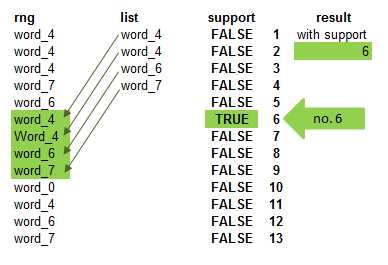With support, the solution is easy. We use this copy-down formula to create the TRUE-FALSE values:`=AND(OFFSET(B2,,,ROWS(list))=list)`Array-entered any copied down, it the four-element blocks of rng with the list - AND ensures that all elements should be equal, so TRUE result occures only in case of the exact match of the pattern. We can find the position of TRUE using MATCH.How can we start to think about this challenge? First of all, compare the elements of rng and list in a matrix: `=--(rng=TRANSPOSE(list))`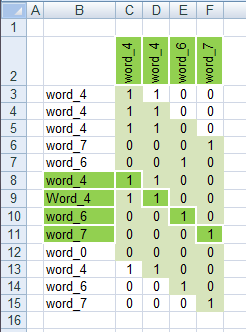If we take into consideration that the order of elements is important, it will be obvious that in case we find the first element of list in rng, the second element must be equal to the next element of rng - in the matrix it is one cell right and one cell below. That means what we are looking for is a “diagonal” pattern in this matrix consists of TRUE(=1) values. So now only need to find where this pattern starts.The idea is to assign a number to each diagonal. Then we only need to count which number appears 4 times (=number of elements of list). We create a simple “marker-matrix” to number the diagonals:`=(ROW(rng)-TRANSPOSE(ROW(OFFSET(rng,,,ROWS(list))))+1)`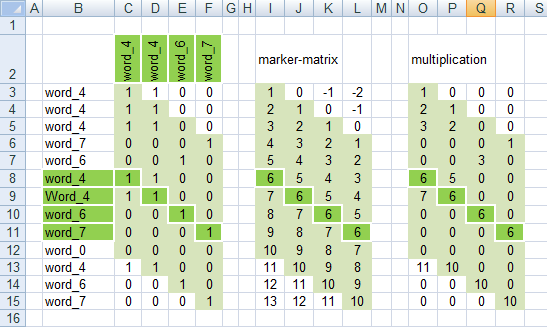After multiplying the elements of the true-false matrix and the marker-matrix, the multiplication will contain exactly 4 equal numbers where the pattern is found in rng. All other values in this matrix is different, because we have the same numbers only in the diagonals. So nothing else is needed: find which number appears 4 times in this matrix!FREQUENCY function will help, we need a vector of numbers starting from 0 (to be able to have correct result if the list starts in the first cell of rng)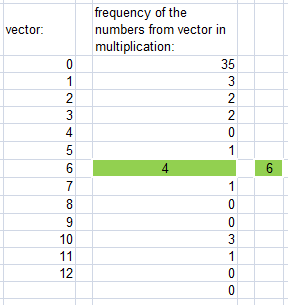Now there are many different ways to find out to which number the correct frequency linked to - the shortest is MATCH, but do not forget to subtract 1 from the result - in the vector we started from 0 :-)The final formula is:`=MATCH(ROWS(list),FREQUENCY((rng=TRANSPOSE(list))*(ROW(rng)-TRANSPOSE(ROW(OFFSET(rng,,,ROWS(list))))+1),ROW(INDIRECT("1:"&(ROWS(rng))))-1),0)-1`There are some different ways to define the marker-matrix and the vector as you can see in the file.The second solution uses a totally different logic. The idea is to create a “pattern code” using MATCH function.We calculate the place of each element of list within list:`=MATCH(list,list,0) -> {1;1;3;4}`And the place of the elements of rng within list:`=MATCH(rng,list,) -> {1;1;1;4;3;1;1;3;4;#N/A;1;3;4}`How can we find the pattern-code within the second MATCH result? The main problem is the different dimension of the two lists and the fact that we need a matrix in which the pattern code of list appears in the diagonal, in all possible starting positions. Like this: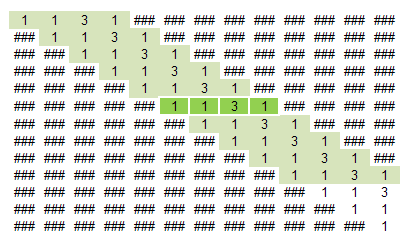Unfortunately it is not possible to use INDEX function in this case, because it could not give a matrix result. So we need some tricks with... SMALL function.This formula will create the above matrix:`=ROUND(MOD(SMALL(ROW(list)+MATCH(list,list,0)%%,TRANSPOSE(ROW(rng))-ROW(rng)+1),1)*10^4,0)`SMALL is capable to give the result in matrix if we put a matrix to it’s second parameter (k). We use a simple constant matrix in which the diagonal elements will do what we need. All the other elements will result an error - as it is visible in the above matrix. We can use IFERROR later in the formula to eliminate these error values.`=TRANSPOSE(ROW(rng))-ROW(rng)+1`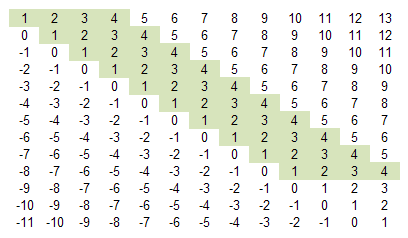The only problem is that we do not need the sorting capability of SMALL, because we need the pattern code in exactly the same order as it is. So we have to add the pattern code to the row numbers as 4th decimal (that is why %% stands in the formula). This way SMALL will not touch the order of the pattern - we only need to cut the decimals from the result.You can see a GREAT! trick in the formula to calculate the decimal part of a number! Using MOD(,1) will do this work beautifully!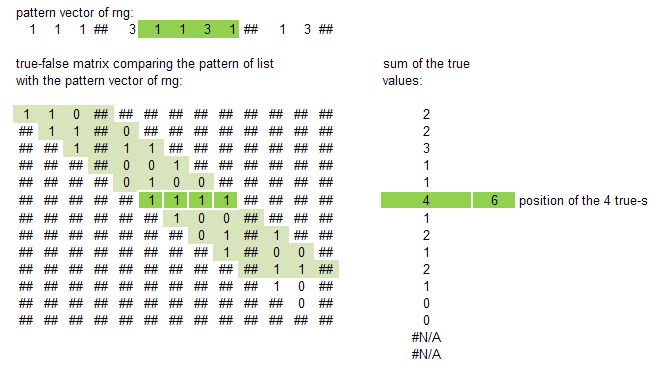Comparing the first matrix with the pattern vector, we have the above true-false matrix, where the 4 true-a appear in the row we are looking for. After summarizing these values with MMULT a simple MATCH formula gives us the exact position number.So the final formula is:`=MATCH(ROWS(list),MMULT(IFERROR(--(TRANSPOSE(MATCH(rng,list,))=ROUND(MOD(SMALL(ROW(list)+MATCH(list,list,0)%%,TRANSPOSE(ROW(rng))-ROW(rng)+1),1)*10^4,0)),0),ROW(rng)^0),)`Words: Kris by FrankensTeamSolutions: Kris, Plinius, r
Ĉ
r,
Nov 13, 2012, 6:40 AM